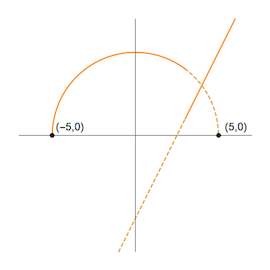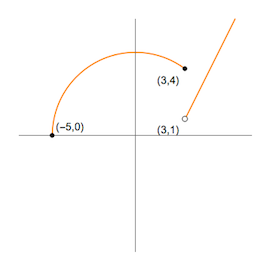## Piecewise Functions

We can create new functions from existing ones in several ways.

When one creates a new function from existing functions in a "piecewise-defined" way, one breaks apart some domain into two or more disjoint pieces, using different functions to calculate the output for each $x$-value, where the function used is based upon the piece into which that particular $x$-value falls.

A simple example of a piecewise defined function is the absolute value function, $|x|$.

When $x \ge 0$, the absolute value function doesn't really do anything -- it returns its input unchanged. So, for this piece $|x| = x$.

However, when $x \lt 0$, the absolute value changes the sign of its input. Multiplying a value by negative one has the same effect -- so we can say that for this second piece $|x| = -x$.

Thus, we summarize how to calculate the output with the following "piecewise definition": $$|x| = \left\{ \begin{array}{ccc} x & , & x \ge 0\\ -x & , & x \lt 0 \end{array} \right.$$

Graphing a piecewise function can be accomplished by simply graphing the functions found in the respective "pieces", limiting the points drawn for each piece to the $x$-values that satisfy the appropriate condition.

For example, given the function $$f(x) = \left\{ \begin{array}{ccc} \sqrt{25-x^2} & , & x \le 3\\ 2x-5 & , & x \gt 3 \end{array} \right.$$

We find its graph by first graphing $y = \sqrt{25-x^2}$ (a semi-circle with center at the origin and radius 5) and $y=2x-5$ (a line of slope 2 with $y$-intercept at $(0,-5)$), as shown at left below. Then, we discard those points that don't match the conditions provided (i.e., the dashed parts of the left graph below), to arrive at the graph of the piecewise-defined function on the right.Note, that we use a filled-in point at $(3,4)$ to suggest that the output of the function at $x=3$ is determined by the semi-circular piece -- since the condition on this piece is true when $x=3$. Likewise, to indicate that the point where $x=3$ should be excluded from the linear piece (given the strict inequality), we place an open circle at $(3,1)$.

The domain of the piecewise-defined function is the set of all $x$ values that: 1) satisfy one of its various conditions, and 2) produce some (real-valued) output. So in the example above, the domain would be $[-5,\infty)$. The range, in a similar way, is the set of all output values (i.e., heights) produced by the function. In the example above, the range would be given by $[0,\infty)$.